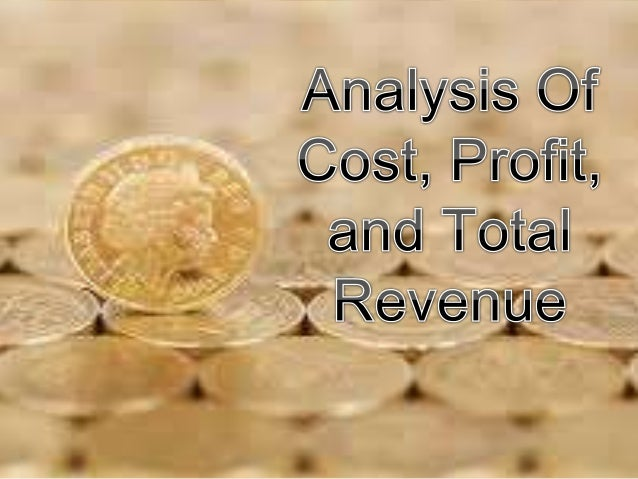Successfully reported this slideshow.
We use your LinkedIn profile and activity data to personalize ads and to show you more relevant ads. You can change your ad preferences anytime.Upcoming SlideShare
×

Analysis of cost, profit, and total revenue

4,037 views

Published on

Analysis of cost, profit, and total revenue

Published in: Economy & Finance
• Full Name
Comment goes here.

Are you sure you want to Yes No
Your message goes here• Be the first to comment

Analysis of cost, profit, and total revenue

1. 1. Cost is the most important consideration in production. A producer will not just jump into a particular investment my simply looking at the potential revenue of the business.
2. 2. The Fundamental concept that should be learned is the difference between economic cost and accounting cost. ECONOMIC COST – are forward looking costs, meaning, economists are in tune with future costs because these costs have major repercussions on the potential profitability of the firm.
3. 3. Accounting Costs – Tend to be retrospective; they recognize cost only when these are made and properly recorded. They do not adjust these costs even if opportunity cost change.
4. 4. - Refers to the actual expenses of the firm in firm in purchasing or hiring the inputs in needs, such as. When a firm purchases a machine worth one million pesos or rents a building worth one hundred thousand pesos per month.
5. 5. – refer to the value of inputs being owned by the firm and used in its own production process.
6. 6. For a firm is a time horizon when one input is held constant. To analyze the short run costs, it is essential to fix the level of capital and study the changes in the quantity of labor hired.
7. 7. 1. Total Fixed Costs (TFC) – These are that do not vary with output. 2. Total Variable Costs( TVC) – These are costs that vary with output. 3. Total Costs (TC) – It is the sum of total fixed costs and total variable costs. Formula: TC – TFC + TVC 4. Average Fixed Cost (AFC) – The total Fixed Costs divided by the number of output produced (Q). Formula: AFC=TFC/Q
8. 8. 5. Average Variable Cost (AVC) – The total Variable costs divided by the number of output produced (Q). Formula: AVC = TVC/ Q 6. Average Total Cost (ATC) – The total cost divided by the number of output produced (Q). It is also defined as the cost per unit of output. Formula: ATC=TC/Q 7. Marginal Cost (MC) – To Changes in total cost divided by the change in output produced (Q). It is also the additional cost incurred from producing additional unit of output. Formula: MC= ∆ TC/ ∆Q 
9. 9. (1) Q (2) TFC (3) TVC (4) TC (5) AFC (6) AVC (7) ATC (8) MC 0 30 O 30 - - - - 1 30 15 45 30 15 45 15 2 30 20 50 15 10 25 5 3 30 22.5 52.5 10 7.5 17.5 2.5 4 30 27.5 57.5 7.5 6.9 14.8 5 5 30 37.5 67.5 6 7.5 13.5 10 6 30 60 90 5 10 15 22.5 Hypothetical Costs Schedules
10. 10. Is the time period wherein all fixed factors can be variable. The long run average total cost of producing a given level of output is always the lowest points of the short run average total cost of producing the output.
11. 11. The Long run Marginal Cost measures the change in long run total cost from a given change in output.
12. 12. A Competitive firm takes the market price as constant. If it wants to maximize profits, the optimum level of production in the short run is when its marginal cost is equal to its price .
13. 13. THE END. THANK YOU FOR LISTENING =)))